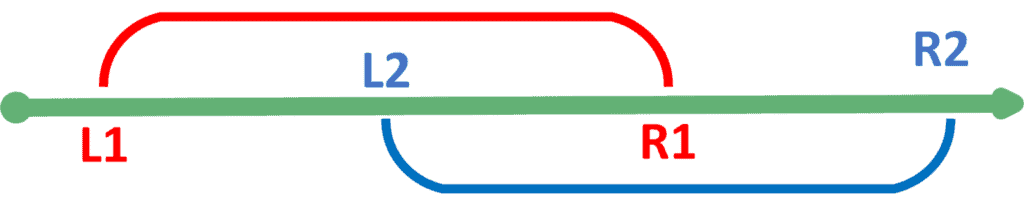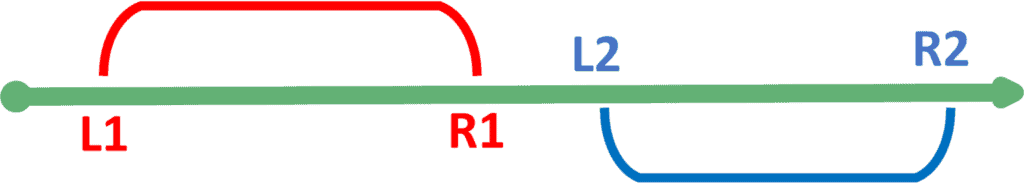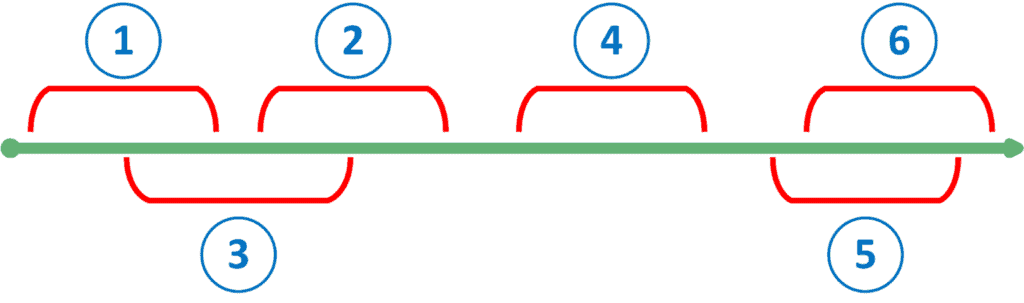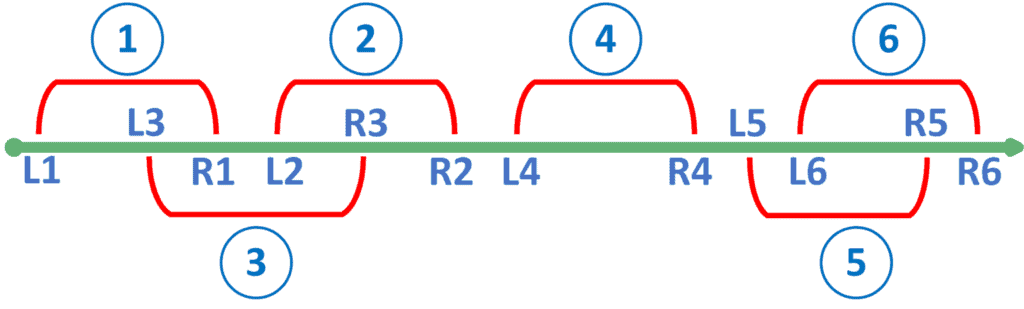## 1. Overview

In this tutorial, we’ll discuss overlapping intervals and how to detect them.

In the beginning, we’ll introduce the problem of finding overlapping ranges. After that, we’ll present two approaches to solving the problem.

## 2. Definitions

Let’s first introduce the concept of overlapping ranges. Then, we can present the problem we discuss in this article.

### 2.1. Overlapping Intervals

Suppose we have a line with fixed points numbered from 1 to. Along this line, we have multiple ranges that cover some parts of this line. We need to define overlapping and non-overlapping intervals.

Let’s take the following overlapping intervals example to explain the idea:If both ranges have at least one common point, then we say that they’re overlapping. In other words, we say that two rangesandare overlapping if:On the other hand, non-overlapping ranges don’t have any points in common. Take a look at the example of the non-overlapping range:We say that two rangesandare non-overlapping if:Now that we have an understanding of what overlapping ranges mean, we can go ahead to explain the problem.

### 2.2. Defining the Problem

In this problem, we’re givenranges. We need to count the number of ranges that are overlapping with at least one more. Even more, we’re asked to return all these overlapping ranges.

Let’s take the following example:The answer to this example is intervals {}. The reason is that each of these intervals overlaps with at least one other interval.

For example, range 3 overlaps with 1 and 2. Similarly, range 5 overlaps with 6.

However, range 4 doesn’t overlap with any other ones. Hence, it’s not presented in the answer.

## 3. Naive Approach

In this approach, we’ll check each interval with all others. Once we find an overlapping, we state that this interval overlaps with some other one and should be added to the answer.

Take a look at the implementation:Firstly, we iterate over all possible ranges. For each range, we iterate over all other ranges to check whether both of them overlap or not. If we managed to find an overlapping, then we add the current one to the answer.

Otherwise, if the current interval doesn’t overlap with any other ones, then we simply continue to the next one.

The complexity of the naive approach is, whereis the number of the intervals.

## 4. Sweep-Line Approach

We’ll explain the theoretical idea of the sweep-line approach and then jump to the implementation of the algorithm.

### 4.1. Theoretical Idea

Suppose we presented all the given intervals on the-axis coordinates. Now, we’ll start fromand move forward. Let’s examine the events we’re going to find on our way.

We have two types of events we could encounter. The first event is the beginning of a certain interval. Similarly, the other event is the end of an interval. However, while moving along the-axis, we need to store the current state.

To do that, we’ll keep the currently open range. By an open range, we mean the one that we found its beginning but didn’t reach its ending yet.

While we’re moving along the-axis, we have two cases.

The first case is that we encounter the beginning of a new interval. If we don’t have any open ranges yet, we simply store the found one as the currently open range. Otherwise, if we have an open range, it means we detected an overlapping. Therefore, we add both the open range and the newly found one to the answer. Also, we need to update the open range we keep.

It’s always optimal to keep the one whose ending is as far to the right as possible. The reason is that it covers a larger distance starting from where we’re standing. Hence, we keep the one that has a larger ending as the currently open interval.

On the other hand, if we encountered the ending of a range, we need to check which range this is. If it’s a different range than the currently open one, we just ignore it. Otherwise, if the current open range is ending, then we clear the currently open range indicating that we don’t have any more open ranges.

### 4.2. Implementation

Let’s take a look at the implementation of the sweep-line approach:First of all, we add all the starting and ending points of each interval to a list. For each interval, we add the point, the type of the point, and its index.

Next, we sort the list. Notice that the sorting of the list should be based on the points. If two elements have the same point, then we must sort them based on the type. Such that, the one whose type is a beginning point comes first. The reason is that since we walk through the elements, we need to discover the beginnings before we discover the endings that might close the currently open interval.

If both the points and the types are equal, we can sort the elements in any order.

After that, we iterate over. If the current element is the beginning of an interval and we don’t have a currently open range, then we store this one as the currently open range. Otherwise, we add the range we found to the answer.

Also, we might add the currently open one to the answer as well. Note that we useto determine whether the current open interval has already been added to the answer or not.

Also, we compare both intervals to store the one that has a larger ending.

However, if we reached the ending of an interval, and it’s the same as the one that is currently open, then we clear the currently open one.

The complexity of the sweep-line approach is, whereis the number of ranges. The complexity is due to the sorting operation.

### 4.3. Example

Let’s take the same example like the one in section 2.2:Let’s list the order of steps for the algorithm:

1. The beginning of interval 1: Since we don’t have any open ranges, we’ll store 1 as the currently open range.
2. The beginning of interval 3: We notice that we have a currently open range. Thus, we add both of them to the answer. Also, we store interval 3 as the currently open one because its ending is larger.
3. The ending of interval 1: It’s not the currently open one. Hence, we ignore it.
4. The beginning of interval 2: We have a currently open range. Therefore, we detect an overlapping and add range 2 to the answer. We don’t add the one with index 3 because it’s already been added. Also, we keep range 2 as the currently open one.
5. The ending of interval 3: We ignore it because it’s not the currently open range.
6. The ending of interval 2: Since it’s the currently open range, we clear it.
7. The beginning of interval 4: Since we don’t have a currently open range, we store range 4 as the open one.
8. The ending of interval 4: It’s the currently open range. So, we clear it.
9. The beginning of interval 5: We don’t have a currently open range. Therefore, we store range 5 as the currently open one.
10. The beginning of interval 6: We detect an overlap. Hence, we add both ranges 5 and 6 to the answer and update the currently open interval to become interval 6.
11. The ending of interval 5: We ignore it because it’s not the currently open one.
12. The ending of interval 6: We clear the currently open range because we reached its ending.

As a result, the answer is {}.

## 5. Conclusion

In this tutorial, we presented overlapping intervals. First of all, we defined overlapping intervals and presented the problem we discuss in this article.

After that, we showed a naive approach to solve the problem.

Finally, we explained the sweep-line approach and provided a step-by-step example that shows how the algorithm actually works.